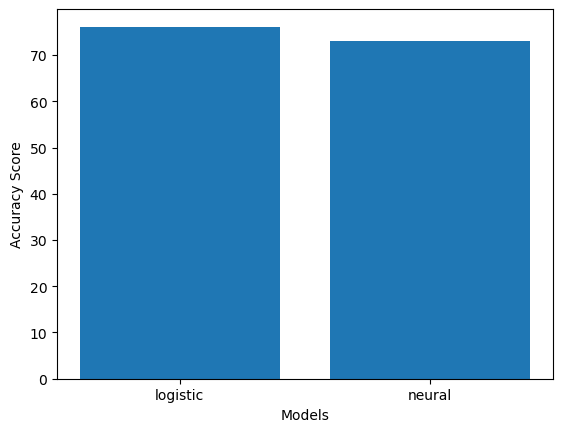In this chapter:

We will do a recap of everything we have learned until now.

## I. Environment Setup

### Step 1: Open the Project folder in VS Code

Go to File –> Open Folder.

### Step 2: Start Julia REPL

Press `Cmd` + `Shift` + `P` (Mac) / `Ctrl` + `Shift` + `P` (Windows), and then search for `Julia: Start REPL`. Hit enter to choose the option from the dropdown menu

### Step 3: Activate Project Environment

• Go to package manager mode. (Press `]` while in Julia REPL to go to Package Manager mode). Then type `activate .` and hit enter. Now you should see your project folder name instead of `(@v1.7) pkg>`.

## Step 4: Create new Julia file for your project

• Go to File –> New File
• Save the file with a name you like. To save the file you can do `Cmd` + `S` / `Ctrl` + `S`.

### Step 5: Make sure you have the required packages

``````using Pkg
Pkg.status()``````
• CSV
• DataFrames
• PyPlot
• ScikitLearn

If some packages are missing, you’ll get an error in the next step.

### Step 6: Load the packages

``````using DataFrames
using CSV
using ScikitLearn
using PyPlot``````

## II. Data

### Step 7: Load the Data

``````pathtodata = joinpath(pwd(), "diabetes.csv")
• Instead of `diabetes.csv`, pass your dataset name.

### Step 8: Inspect the data columns

``first(data,5)``

5 rows × 8 columns

npregglubpskinbmipedagetype
Int64Int64Int64Int64Float64Float64Int64String3
16148723533.60.62750Yes
2185662926.60.35131No
3189662328.10.16721No
4378503231.00.24826Yes
52197704530.50.15853Yes
• If you find columns that are redundant or meaningless, delete those columns. For example, suppose we had a meaningless column called `RowNo`. To delete the column you can use the command `data = select(data,Not(:RowNo))`. Make sure you only run the delete command once per column. Trying to run it multiple times will give you an error.

### Step 9: Extract the features and target

``````X = Array(data[!,Not(:type)]);
y = Array(data[!, :type]);``````
• Instead of `:type` in the above code, you should use the column name you have for your target.

## III. Model Training

### Step 10: Split the data into training set and test set.

``````@sk_import model_selection: train_test_split;
X_train, X_test, Y_train, Y_test =
train_test_split(X, y, test_size=0.2,
random_state=42);``````
• Here I chose 20% for test set (so 80% of the actual data will be used for training).

### Step 11: Build a baseline model

Before going into complicated models, it’s recommended that you try a simple model first. You’ll call this your baseline model. In our case, we’ll have a simple logistic regression model our baseline.

``````@sk_import linear_model: LogisticRegression;
simplelogistic =LogisticRegression();

fit!(simplelogistic, X_train, Y_train);``````

### Step 12: Check the accuracy of your baseline model

``````@sk_import metrics: accuracy_score

Y_pred_train = predict(simplelogistic,X_train);
print(accuracy_score(Y_train,Y_pred_train))``````
``0.7886792452830189``

### Step 13: Create a slightly complicated model and check its accuracy

We’ll have a shallow neural network with one hidden layer and 5 nodes as our slightly complicated model.

``````@sk_import neural_network: MLPClassifier;
simpleneuralnetwork = MLPClassifier(hidden_layer_sizes=(5));

fit!(simpleneuralnetwork, X_train, Y_train);

Y_pred_train = predict(simpleneuralnetwork,X_train);
print(accuracy_score(Y_train,Y_pred_train))``````
``0.30943396226415093``

### Step 14: Search the model space for better hyperparameters with 3-Fold cross validated training

``````using ScikitLearn.GridSearch: GridSearchCV
gridsearch_logistic = GridSearchCV(LogisticRegression(),
Dict(:solver => ["newton-cg", "lbfgs", "liblinear"],
:C => [0.01, 0.1, 0.5, 0.9]))

fit!(gridsearch_logistic, X_train, Y_train);``````
• `:C` is a regularization parameter.
• For more hyperparameters that are tweakable, please refer to LogisticRegression’s ScikitLearn documentation page.

### Step 15: Repeat GridSearchCV for other models you have

• Just don’t simply use the list of numbers I have used for the `hidden_layer_sizes`. It might make sense to have 1000s of nodes and many hidden layers if you are doing image processing.

Sometimes, you might have to do multiple grid search rounds with different hyperparameter settings to find the most optimal values.

``````gridsearch_neuralnet = GridSearchCV(MLPClassifier(),
:hidden_layer_sizes => [(2), (20), (1,5,10), (10,10), (10,20,10)]))
fit!(gridsearch_neuralnet, X_train, Y_train);

gridsearch_neuralnet_results = DataFrame(gridsearch_neuralnet.grid_scores_);
hcat(DataFrame(gridsearch_neuralnet_results.parameters),
gridsearch_neuralnet_results)[!,Not(:parameters)]``````

15 rows × 4 columns

solverhidden_layer_sizesmean_validation_scorecv_validation_scores
StringAnyFloat64Array…
1sgd20.562264[0.685393, 0.306818, 0.693182]
2lbfgs20.690566[0.685393, 0.693182, 0.693182]
4sgd200.686792[0.662921, 0.693182, 0.704545]
5lbfgs200.709434[0.730337, 0.681818, 0.715909]
7sgd(1, 5, 10)0.543396[0.674157, 0.647727, 0.306818]
8lbfgs(1, 5, 10)0.690566[0.685393, 0.693182, 0.693182]
10sgd(10, 10)0.660377[0.662921, 0.693182, 0.625]
11lbfgs(10, 10)0.716981[0.707865, 0.693182, 0.75]
13sgd(10, 20, 10)0.683019[0.58427, 0.715909, 0.75]
14lbfgs(10, 20, 10)0.750943[0.707865, 0.772727, 0.772727]
``best_neuralnetwork_model = gridsearch_neuralnet.best_estimator_``
``PyObject MLPClassifier(hidden_layer_sizes=(10, 20, 10), solver='lbfgs')``

### Step 16: Compare the results of competing models on test set

``````@sk_import metrics: accuracy_score
Y_pred_test_logistic = predict(best_logistic_model, X_test)
logistic_accuracy = accuracy_score(Y_test,Y_pred_test_logistic)

Y_pred_test_neural = predict(best_neuralnetwork_model, X_test)
neural_accuracy = accuracy_score(Y_test,Y_pred_test_neural)

models = ["logistic","neural"]
scores = [logistic_accuracy, neural_accuracy]
# Plotting the results

using PyPlot
figure()
b = PyPlot.bar(x = models, height = scores*100);
xlabel("Models");
ylabel("Accuracy Score"); ``````Here we have used accuracy for comparison. You can also use precision, recall, or f1 score in a similar fashion for comparing models, depending on the domain of prediction.

### Step 17: Save your best model for production

The model you found to be the best performing one can be saved to the disk so that you don’t have to train your models every time you restart Julia or you want to make predictions. We’ll need three packages to save a `scikit-learn` model: `PyCall`, `PyCallJLD`, and `JLD`

``````using PyCall, JLD, PyCallJLD
save("saved_file.jld", "diabetic_prediction", best_logistic_model)``````
• The save function takes three arguments: the name of the file, the name you want to give to the model, the trained model.

To load a saved `scikit-learn` model, you can use the load function:

``logistic_model = load("saved_file.jld", "diabetic_prediction")``
``PyObject LogisticRegression(C=0.01, solver='newton-cg')``

Now let’s check if our loaded model is working.

``predict(logistic_model, X_test)[1:4]``
``````4-element Vector{Any}:
"No"
"No"
"No"
"No"``````